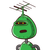# A rare whale fossil was uncovered under layers of sediment. When the excavator took measurements, she found the tail to be as long

A rare whale fossil was uncovered under layers of sediment. When the excavator took measurements, she found the tail to be as long as its head plus a quarter of the length of the body. When the archaeologist came in, he measured the length of the body. He found it to be ¾ of the total length. They made arguments about how such a fish would swim in the water. To make proper predictions they measured the head size, and found it to be 4 inches long. What is the total length of this whale?

128

99

136

142

with proof

no sPams

spammers will be prosecuted ​

1.Let us assume tail to be t, head to be h, length of body be x and the total length be L.

Tail = Head + ¼ of length of body

→ t = h + ¼ x

Length of body = ¾ of total length

→ x = ¾ L

Also,

Total length = Length of body + Head + tail

→ L = x + h + t

Now, the question has given us the length of head. Substituting the value :-

$$\implies\sf t = 4 + \dfrac{1}{4}x \: \: \: \: \: \: -i$$

$$\implies\sf x = \dfrac{3}{4} L \: \: \: \: \: \: -ii$$

$$\implies\sf L = x + 4 + t \: \: \: \: \: \: -iii$$

Substituting the value of t from equation i in equation iii :-

$$\implies\sf L = x + 4 + t$$

$$\implies\sf L = x + 4 + 4 + \dfrac{1}{4}x$$

$$\implies\sf L = 8 + x + \dfrac{1}{4}x\: \: \: \: \: \: -iv$$

Substituting the value of x from equation ii in equation iv :-

$$\implies\sf L = 8 + \dfrac{3}{4} L + \dfrac{1}{4} \times \dfrac{3}{4} L$$

$$\implies\sf L = 8 + \dfrac{3}{4} L + \dfrac{3}{16} L$$

$$\implies\sf L = 8 + \dfrac{12}{16} L + \dfrac{3}{16} L$$

$$\implies\sf L = 8 + \dfrac{15}{16} L$$

$$\implies\sf L – \dfrac{15}{16} L = 8$$

$$\implies\sf \dfrac{1}{16} L = 8$$

$$\implies\sf L = 8 \times 16$$

$$\implies\sf L = 128$$

### Verification :-

From equation ii :-

$$\implies\sf x = \dfrac{3}{4}L$$

$$\implies\sf x = \dfrac{3}{4} \times 128$$

$$\implies\sf x = 96$$

Substituting the value in equation i :-

$$\implies\sf t = h + \dfrac{1}{4} x$$

$$\implies\sf t = 4 + \dfrac{1}{4} \times 96$$

$$\implies\sf t = 4 + 24$$

$$\implies\sf t = 28$$

Substituting the value of t , x , h and L in equation iii :-

$$\implies\sf L = t + x + h$$

$$\implies\sf LHS \longrightarrow L = 128$$

$$\implies\sf RHS \longrightarrow t + x + h$$

$$\implies\sf RHS = 28 + 96 + 4$$

$$\implies\sf RHS = 128$$

LHS = RHS

Hence verified.